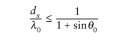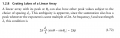# basic avoing grating lobe formula logic

#### yef smith

Joined Aug 2, 2020
348
hello,I have this formula bellow expression 1.72
p=1 (grating lobe of the first order) and we have expression 1 shown bellow.
we have the condition for no grating lobe called expression 2.
i know the sine is smaller then 1 but why is that a guarntee that no grating lobe will accure?
Thanks.
expression 1:
$\frac{d}{\lambda}=\frac{1}{sin(\theta)-sin(\theta_0)}$

expression 2: